Primary Math

# Primary MathFinding the Size of an Angle in a Triangle given the Other Two Angles Sizes Education-MathAn Easy Complex Way to Find the Treasure | Popular Maths | Nagwa Education-Math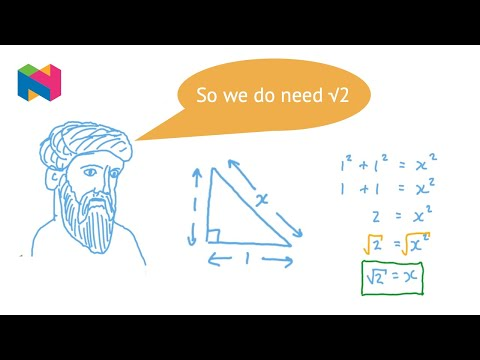Proving that the Square Root of Two is Irrational Education-MathHow to multiply two fractions Education-MathFinding Sides Using the Sine Ratio | Trigonometry | Nagwa Education-MathFinding the Areas of Sectors of Circles Education-MathApplications of Trigonometric Ratios | Trigonometry | Nagwa Education-Math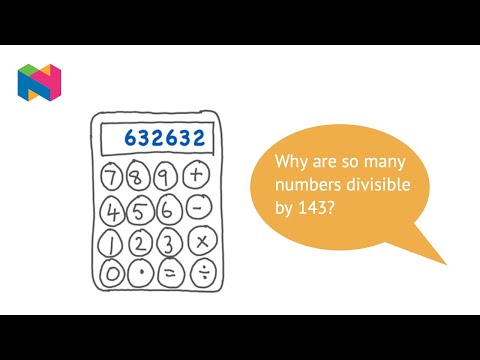The Suprising Divisibility of Numbers | Popular Maths | Nagwa Education-MathArea of Triangles Education-MathThe Monty Hall Problem | Popular Maths | Nagwa Education-MathTrigonometric Ratios for Complementary Angles | Trigonometry | Nagwa Education-MathConverting Fractions to Percentages Education-MathSimplifying Fractions Education-MathComparing Fractions with the Same Numerator or Denominator Education-MathThe Triangle Inequality Education-MathFinding the Area of a Triangle given Its Dimensions Education-MathUsing Tangents | Trigonometry | Nagwa Education-MathSolving Cryptarithmetic Puzzles | Popular Maths | Nagwa Education-MathTrigonometric Ratios | Trigonometry | Nagwa Education-MathAreas of Circles Education-Math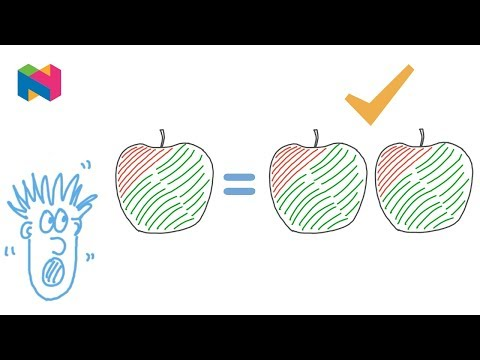1 equals 2 | Popular Maths | Nagwa Education-MathParallel Lines and Transversals Education-MathDividing Fractions and Mixed Numbers Education-MathFinding the Percent of a Quantity with Simple Fractions and Integers Education-MathInterior Angles of a Regular Polygon"},"params":{"allowfullscreen":"true Education-Math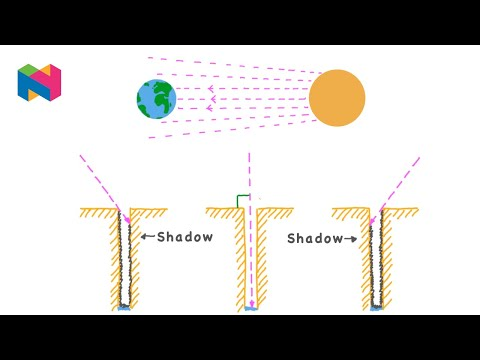Eratosthenes Measures the Circumference of the Earth Education-MathThe Pythagorean Theorem Distance between Two Points | Trigonometry | Nagwa Education-Math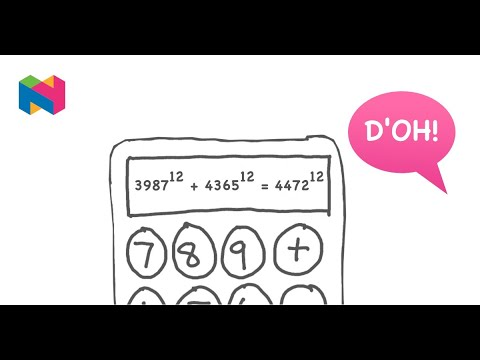Fermats Last Theorem | Popular Maths | Nagwa Education-Math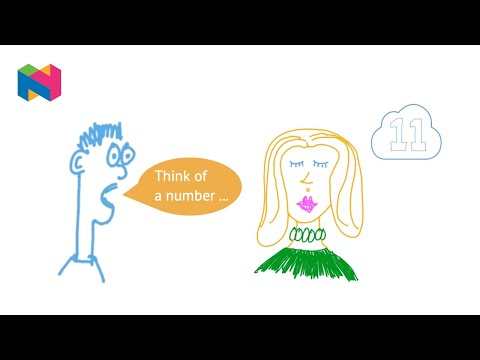Mind Reading Number Trick | Popular Maths | Nagwa Education-Math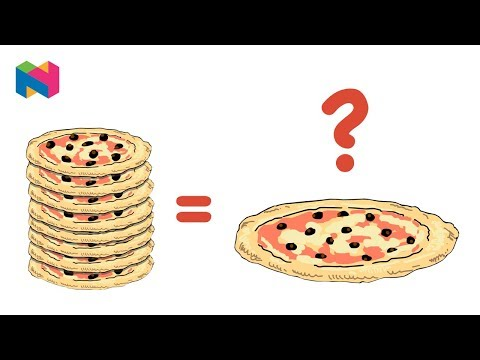How Scale Factor Maths Upset My Pizza Night | Popular Maths | Nagwa"}};ytplayer.load = function() {yt.player.Application.create("player-api Education-MathFinding the Sum of the Interior Angles of a Hexagon Education-MathRecognize and Generate Simple Equivalent Fractions Education-MathAn Angle between a Line and the x-Axis | Trigonometry | Nagwa Education-MathConverting Fractions to Decimals Education-MathConverting Percentages to Fractions Education-MathFinding an Unknown Side Length in a Trapezium Using Pythagorass Theorem Education-MathThe Pythagorean Theorem Introduction | Trigonometry | Nagwa Education-MathAngles in Triangles Education-MathUnit Fractions Education-MathAngle Rules Education-MathSum of the Interior Angles of a Polygon Education-MathSolving Word Problems Involving Subtraction of Fractions with Unlike Denominators Education-MathGarfields Proof of the Pythagorean Theorem | Trigonometry | Nagwa Education-MathDividing Whole Numbers by Fractions in Word Problems Education-Math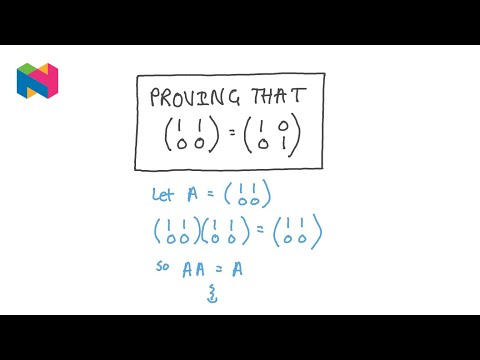Fake Proof that Matrix 𝐴 = Matrix 𝐼 | Popular Maths | Nagwa Education-MathUsing the Area and Circumference of a Circle Education-MathFinding the Length of a Side in a Right Angled Triangle Using the Pythagorean Theorem Education-MathFinding Angles Using the Sine Ratio | Trigonometry | Nagwa Education-Math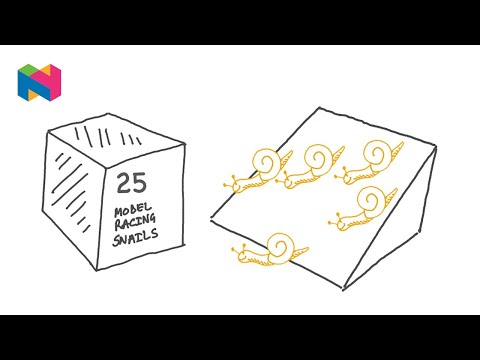Snail Racing Logic Puzzle | Popular Maths | YouTube Education-MathConverting Improper Fractions to Mixed Numbers Education-MathAdding and Subtracting Fractions with Common Denominators Education-MathFinding the Areas of Circles Education-MathFinding Angles Using the Cosine Ratio | Trigonometry | Nagwa Education-MathFinding Sides Using the Cosine Ratio | Trigonometry | Nagwa"},"url":" Education-MathUsing the Properties of Parallel Lines to Solve a Problem Education-MathAngles of Elevation and Depression | Trigonometry | Nagwa Education-MathExpress Whole Numbers as Fractions and Recognise Fractions that are Whole Numbers Education-MathRepresenting Fractions on the Number Line Education-MathAdd and Subtract Unlike Fractions Using Least Common Multiple Education-MathThe Pythagorean Theorem Example Question Education-MathUsing Diagrams to Compare Fractions with Different Denominators Education-MathFinding in the Simplest Form the Result of Dividing Two Fractions Education-MathArc Length and Area of Sector Education-Math

X